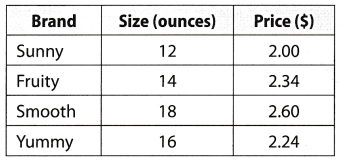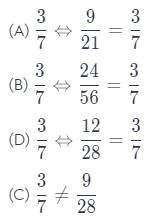# Texas Go Math Grade 6 Benchmark Test Answer Key Part 1

Refer to our Texas Go Math Grade 6 Answer Key Pdf to score good marks in the exams. Test yourself by practicing the problems from Texas Go Math Grade 6 Benchmark Test Answer Key Part 1.

## Texas Go Math Grade 6 Benchmark Test Answer Key Part 1

Selected Response

Question 1.
Which temperature is coldest?
(A) – 13° F
(B) 20° F
(C) -20° F
(D) 13° F
(C) -20°F

Explanation:
The smallest number of all, of those is -20, so, we can conclude that the coldest temperature is -20° F.

Question 2.
Which group of numbers is in order from least to greatest?
(A) 2.58, 2$$\frac{5}{8}$$, 2.6, 2$$\frac{2}{3}$$
(B) 2$$\frac{2}{3}$$, 2$$\frac{5}{8}$$, 2.6, 2.58
(C) 2$$\frac{5}{8}$$, 2$$\frac{2}{3}$$, 2.6, 2.58
(D) 2.58, 2.6, 2$$\frac{5}{8}$$, 2$$\frac{2}{3}$$
(D) 2.58, 2.6, 2$$\frac{5}{8}$$, 2$$\frac{2}{3}$$

Explanation:
The group D is in required order because:
2$$\frac{5}{8}$$ = 2.625
2$$\frac{5}{8}$$ = 2.667
2.58 < 2.6 < 2.625 < 2.667

Question 3.
Evaluate a + b for a = -46 and b = 34.
(A) -12
(B) 80
(C) -80
(D) 12
(A) -12

Explanation:
We will, substitute 46 for a and 34 for b in expression a + b in order to evaluate it, so, we have the following:
46 + 34= 12

Question 4.
One winter day, the temperature ranged from a high of 40°F to a low of -5°F. By how many degrees did the temperature change?
(A) 45° F
(B) 35° F
(C) 25° F
(D) 55° F
(A) 45° F

Explanation:
In order to find how many degrees the temperature changed, we need to subtract those two values, and get:
40 – (-5) = 40 + 5
= 45
So, the temperature changed for 45° F

Question 5.
Find the quotient. 9$$\frac{3}{5}$$ ÷ $$\frac{8}{15}$$
(A) 2
(B) 18
(C) 16$$\frac{7}{8}$$
(D) 18$$\frac{3}{4}$$
(B) 18

Explanation:
In order to find the final quotient we need to simplify given expression:
$$\frac{48}{5}$$ ∙ $$\frac{15}{8}$$ = $$\frac{6}{1}$$ ∙ $$\frac{3}{1}$$
= 18
So, the answer is that result is (B) 18.

Question 6.
Juan purchased 3.4 pounds of nails at a cost of $4.51 per pound. What was the cost of the nails? (A)$153.34
(B) $1.53 (C)$7.91
(D) $15.33 Answer: (D)$15.33

Explanation:
To find what was the cost of the nails, we need to multiply those values. So, we have the following:
3.4 ∙ 4.51 = 15.33
So, the cost of the nails was $15.33. Question 7. You are working as an assistant to a chef. The chef has 6 cups of berries and will use $$\frac{2}{3}$$ cup of berries for each dessert he makes. How many desserts can he make? (A) 4 desserts (B) 6$$\frac{2}{3}$$ desserts (C) 9 desserts (D) 12 desserts Answer: (C) 9 desserts Explanation: If we want to find how many deserts the chef can make, we need to divide 6 by $$\frac{2}{3}$$. So, we have the following: 6 : $$\frac{2}{3}$$ = 6 ∙ $$\frac{3}{2}$$ = $$\frac{18}{2}$$ = 9 So, correct answer is C, he can make 9 desserts. Question 8. Jorge is building a table out of boards that are 3.75 inches wide. He wants the table to be at least 36 inches wide. How many boards does he need? (A) 9 (B) 9.6 (C) 10 (D) 135 Answer: (B) 9.6 Explanation: In order to find how many boards Jorge needs for table to be at least 36 inches, we need to divide 36 by 3.75 and get: 36 ÷ 3.75 = 9.6 So, he needs 9.6 boards. Question 9. The fuel for a chainsaw is a mix of oil and gasoline. The label says to mix 6 ounces of oil with 16 gallons of gasoline. How much oil would you use if you had 24 gallons of gasoline? (A) 3 ounces (B) 9 ounces (C) 12 ounces (D) 85.3 ounces Answer: (B) 9 ounces Explanation: Because, according to label, it is mixed 6 ounces of oil with 16 gallons of gasoline, we need to calculate x, which is oil in ounces needed for mix with 24 gallons of gasoline So, we have the following: 6 → 16 x → 24 x = $$\frac{6 \cdot 24}{16}$$ = 9 So, conclusion is that it is 9 ounces of oil needed for 24 gallons of gasoline. Question 10. A grocery store sells the brands of yogurt shown in the table.Which brand of yogurt has the lowest unit price? (A) Sunny (B) Fruity (C) Smooth (D) Yummy Answer: (B) Fruity Explanation: In order to find the lowest unit price, we need to find quotient between size and price for each brand of yogurt Sunny: $$\frac{12}{2}$$ = 6 Fruity: $$\frac{14}{2.34}$$ = 5.98 Smooth: $$\frac{18}{2.6}$$ = 6.92 Yummy: $$\frac{16}{2.24}$$ = 7.14 We can conclude that the lowest unit price has fruity yogurt. So, correct answer is B. Question 11. Find the unit rate. Patricia paid$385 for 5 nights at a hotel.
(A) $$\frac{\ 77}{1 \text { night }}$$
(B) $$\frac{\ 154}{1 \text { night }}$$
(C) $$\frac{\ 385}{1 \text { night }}$$
(D) $$\frac{\ 39}{1 \text { night }}$$
(A) $$\frac{\ 77}{1 \text { night }}$$

Explanation:
In order to find the unit rate we have to divide $35 which is the sum of money Patricia paid for 5 nights by 5. On that way we will find how much costs one day: $$\frac{385}{5}$$ = 77 So, Patricia paid$ 77 for one night and required unit rate is $$\frac{\ 77}{1 \text { night }}$$.

Question 12.
Which of the following does NOT show a pair of equivalent ratios?
(A) $$\frac{3}{7}$$, $$\frac{9}{21}$$
(B) $$\frac{24}{56}$$, $$\frac{3}{7}$$
(C) $$\frac{3}{7}$$, $$\frac{9}{28}$$
(D) $$\frac{3}{7}$$, $$\frac{12}{28}$$
(C) $$\frac{3}{7}$$, $$\frac{9}{28}$$

Explanation:
We can see that only at C we do not have pair of equivalent ratios.Question 13.
Gina paid $129 for a bicycle that was on sale for 75% of its original price. What was the original price of the bicycle? (A)$54.00
(B) $96.75 (C)$172
(D) $204 Answer: (C)$172

Explanation:
First we need to form next proportion in order to find the originaL price of the bicycle:
x = $$\frac{129 \cdot 100}{75}$$ = $172 So, conclusion is$172 was the original price of the bicycle.

Question 14.
Write the fraction $$\frac{9}{50}$$ as a percent. If necessary, round your answer to the nearest hundredth.
(A) 0.18%
(B) 0.45%
(C) 18%
(D) 45%
(C) 18%

Explanation:
If we want to write given fraction as a percent, we need multiply it by 100 and get:
$$\frac{9}{50}$$ ∙ 100 = $$\frac{900}{50}$$ = 18
So, $$\frac{9}{50}$$ is 18%. Correct answer is C.

Question 15.
Write an equation you can use to find the missing value in the table.(A) t = a+1
(B) t = a + 15
(C) t = a + 3
(D) t = a + 10
(C) t = a + 3

Explanation:
From the table we can conclude that we can use equation C, that is t = a + 3, in order to find missing value it the table.

Question 16.
Which expression is NOT equivalent to the expression 38 – 14?
(A) 2(19 – 7)
(B) 2(19) – 2(7)
(C) (19 – 7)2
(D) 2(36 – 12)
(D) 2(36 – 12)

Explanation:
We can notice the following:
2(19 – 7) = (19 – 7)2
= 2(19) – 2(7)
= 38 – 14
Also, we can see that only D is not equivalent to the expression 38 – 14.

Question 17.
Suppose you have developed a scale that indicates the brightness of sunlight. Each category in the table is 9 times brighter than the next category. For example, a day that is dazzling is 9 times brighter than a day that is radiant. How many times brighter is a dazzling day than an illuminated day?(A) 2 times brighter
(B) 9 times brighter
(C) 81 times brighter
(D) 729 times brighter
(C) 81 times brighter

Explanation:
Because each category in the table is 9 times brighter than the next category and we already have that dazzling day is 9 times brighter than radiant day, and we can conclude that radiant day is 9 times brighter than a day that is illuminated, the conclusion is that dazzling day is 9 ∙ 9 = 81 times brighter than an illuminated day.

Question 18.
Evaluate the expression. (28 ÷ 4) ∙ 5 – 6 + 42
(A) 9
(B) 13
(C) 37
(D) 45
(D) 45

Explanation:
In this expression first we caLcuLate part of it which is in brackets and squating, then muLtipLy, add or subtract at the end.
So, we have the following:
7.5 – 6 + 42 = 35 – 6 + 16
= 29 + 16
= 45
Conclusion is that result is 45, so, correct answer is D.

Gridded Response

Question 19.
Shari bought 2$$\frac{1}{2}$$ yards of fabric for $7.99 per yard. She used $$\frac{3}{4}$$ of the fabric to make decorative pillows. How much money could Shari have saved by only buying the amount of fabric that she used? Write your answer in dollars and round to the nearest tenth.Answer: For 2 ∙ $$\frac{1}{2}$$ yards of fabric, Shari paried: 2$$\frac{1}{2}$$ ∙ 7.99 = $$\frac{5}{2}$$ ∙ 7.99 = 19.975 But , she used only $$\frac{3}{4}$$, and for $$\frac{3}{4}$$ yards of fabric, she would pay: $$\frac{3}{4}$$ ∙ 7.99 = 5.9923 So. she could save 19.975 – 5.9923 ≈$14.

Scroll to Top